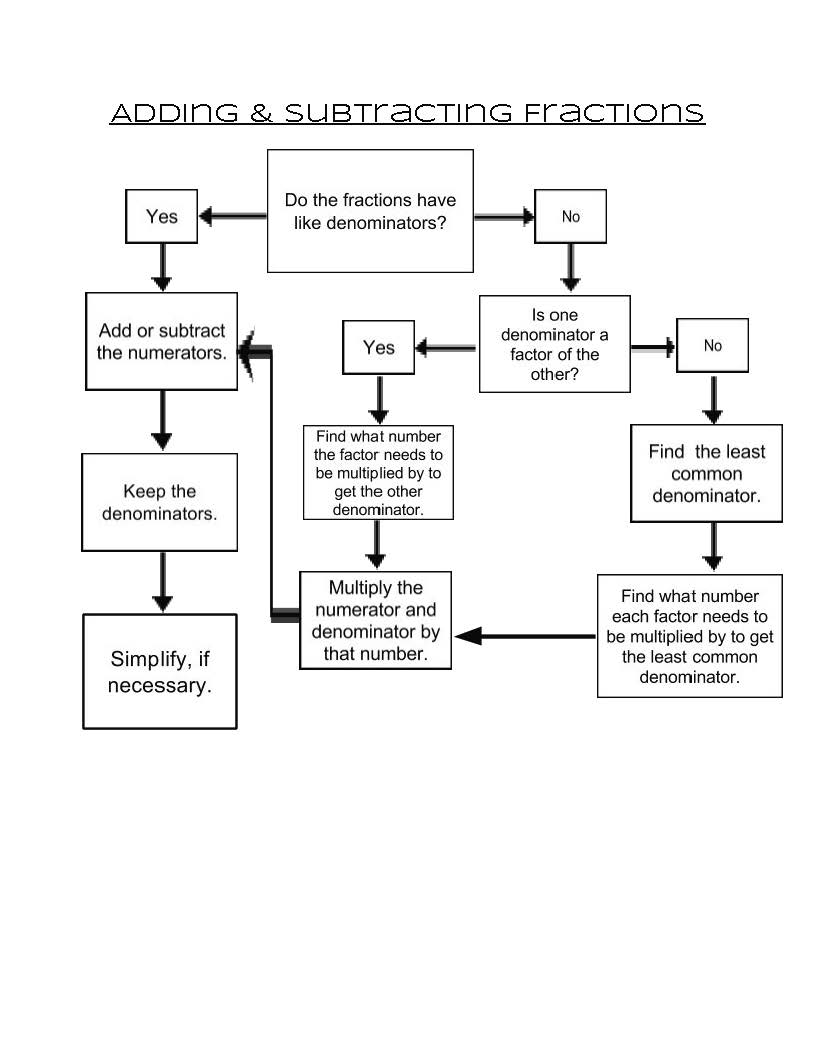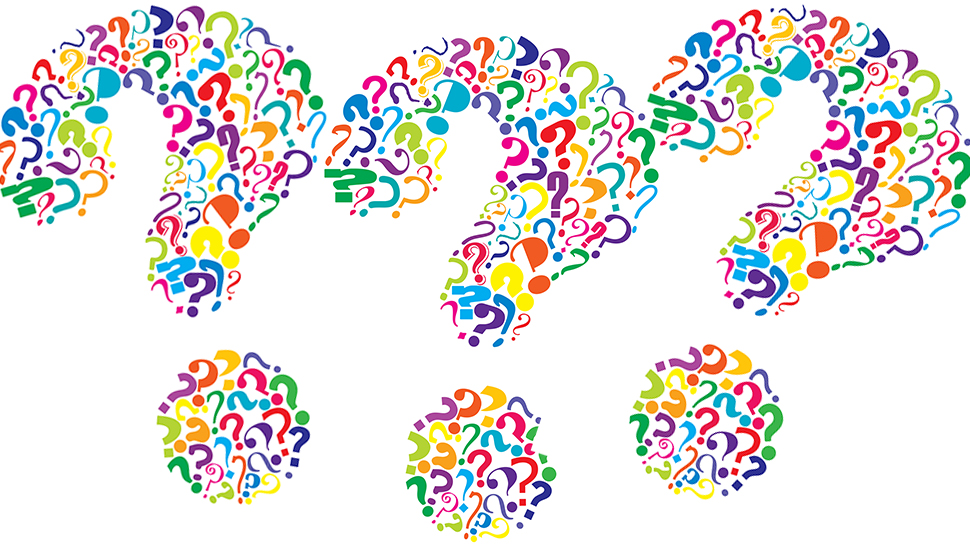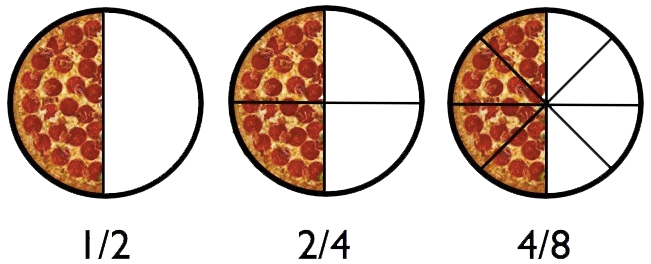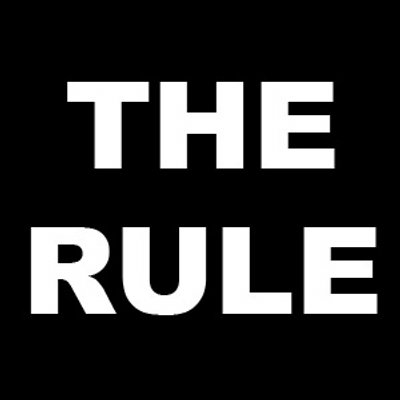starstarstarstarstarstarstarstarstarstar
by Rianna Daughtry
| 12 Questions
Lesson Essential Question

What are the characteristics of fractions that can be added or subtracted?Vocabulary Terms
• Like Fractions: fractions with the same denominator
• Unlike Fractions: fractions with different denominators
• Numerator: the number of parts represented in a fraction; the top number
• Denominator: the number of parts in the whole; the bottom number
• Least Common Denominator: the LCM (least common multiple) of the denominators of the fractions
• Greatest Common Factor: the greatest of the common factors of two or more numbers
• Equivalent Fractions: fractions that name the same number
• Simplest Form: the form of a fraction where the GCF (greatest common factor) of the numerator and the denominator is 1.
Graphic Organizer

Below is a graphic organizer on adding and subtracting fractions. It breaks down the characteristics of fractions and the procedural steps that one must take when dealing with "like" and "unlike fractions. This is to be used as a guide and reference throughout the duration of the lesson.

***If you would prefer to have a physical copy of the graphic organizer, please see the teacher to receive one***What is the difference between like and unlike fractions?

Take a look at the video below. This video is helpful to understand the concept of like and unlike fractions using easy and appropriate examples such as students in a classroom.
1
1
"Unlike" fractions are fractions with different denominators. True or False?
True
False
2
1
Which of the following fraction sequences consist of ALL "unlike" fractions?
1/9, 3/9/, 5/9, 7/9
1/6, 2/5, 4/7, 3/8
1/2, 3/4, 1/4, 3/2
3
1
What makes a fraction a "like" fraction?
The denominators are different.
The numerators are the same.
The denominators are the same.
4
1
The following fraction sequences are "like" fractions. Select all that apply.
1/2, 3/2, 5/2, 7/2
2/6, 4/8, 7/3, 5/4
3/8, 4/8, 5/8, 7/8

# Adding and subtracting LIKE fractions

Like fractions are fractions with the same denominator. You can add and subtract like fractions easily - simply add or subtract the numerators and write the sum over the common denominator.5
1
Find the sum of (7/10) + (9/10). Show ALL of your work.
6
1
Find the sum of (6/15) + (7/15). Show ALL of your work.
7
1
Find the difference of (8/9) - (3/9). Show ALL of your work.
8
1
Find the difference of (16/19) - (9/19). Show ALL of your work.

# What are equivalent fractions?

Equivalent fractions are fractions which have the same value, even though they may look different.
For example, 1/2 and 2/4 are equivalent, because they are both "half". See the illustration below.Take a look at the video below. The video explains and demonstrates how to make "unlike" fractions into equivalent fractions by determining the leacst common denominator. Stop the video at 3:49 and move on to the next topic/activity.# The Equivalent Fraction Rule:

Equivalent fractions can ONLY be made by multiplying or dividing AND whatever is done to the numerator (top), has got to be done to the denominator (bottom).
Take a look at the video below entitled "Adding Fractions with Different Denominators". The video reviews how to add "like" fractions and then demonstrates how to make equivalent fractions of "unlike" fractions using the least common denominator. Stop the video at 3:27 and answer the questions below.9
1
Equivalent fractions can ONLY be made by adding and subtracting AND whatever is done to the numerator, has got to be done to the denominator.
True
False
10
1
Which is the equivalent fraction of (3/4)?
5/4
2/3
1/9
6/8
11
1
Find the sum of (4/5) + (9/10). Be sure to show ALL work.
12
1
Find the difference of (10/12) - (1/4). Be sure to show ALL work.